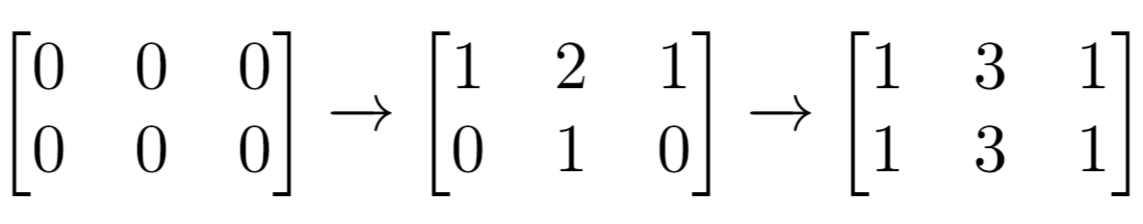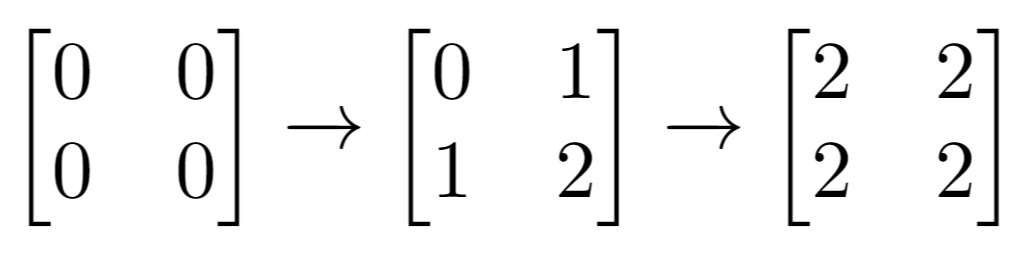### LeetCode - Arrays - Cells with Odd Values in a Matrix

Given `n` and `m` which are the dimensions of a matrix initialized by zeros and given an array `indices` where `indices[i] = [ri, ci]`. For each pair of `[ri, ci]` you have to increment all cells in row `ri` and column `ci` by 1.

Return the number of cells with odd values in the matrix after applying the increment to all `indices`.

Example 1:```Input: n = 2, m = 3, indices = [[0,1],[1,1]]
Output: 6
Explanation: Initial matrix = [[0,0,0],[0,0,0]].
After applying first increment it becomes [[1,2,1],[0,1,0]].
The final matrix will be [[1,3,1],[1,3,1]] which contains 6 odd numbers.
```

Example 2:```Input: n = 2, m = 2, indices = [[1,1],[0,0]]
Output: 0
Explanation: Final matrix = [[2,2],[2,2]]. There is no odd number in the final matrix.```

```class Solution {     public int oddCells(int n, int m, int[][] indices) {         int[][] matrix = new int[n][m];         for(int i=0;i<n;i++){             for(int j=0;j<m;j++){                 matrix[i][j] = 0;             }         }                  for(int[] index: indices){             int row = index;             int col = index;             for(int c=0;c<m;c++){                 matrix[row][c]++;             }             for(int r=0;r<n;r++){                 matrix[r][col]++;             }         }         int counter = 0;         for(int i=0;i<n;i++){             for(int j=0;j<m;j++){                 if(matrix[i][j]%2 != 0) counter++;             }         }                  return counter;         } }```